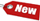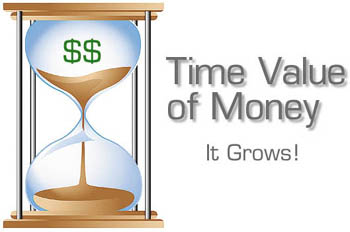Browse Accounting Lessons HereAccounting Terms & DefinitionsAccounting for Merchandising ActivitiesDebits and Credits (Double Entry Accounting)Business Valuation FormulasTime Value of Money & Present/Future ValuesComplex Debt & Equity InstrumentsCommon Stock & Shareholder's EquityAccounting & Finance RatiosValuing Common StockCorporate Income TaxesLower of Cost or Market (LCM) & Inventory ValuationChart of Accounts & BookkeepingBonds Payable & Long Term LiabilitiesCapital AssetsGAAP, Accrual & Cash Accounting, Information Commodity, Internal Controls & MaterialityWhat category of browser are you on this website? Accounting student (homework help) Finance professor (university research) Accounting manager (at work) Other Explore Careers in Accounting and FinanceVisit our section on Careers in Accounting & Finance to explore vast opportunities in this industry.

Chapter 4.1 ® - Time Value of Money, Future Values of Compounding Interest, Investing for more than 1 Period & Examination of Original Investment & Growth of InvestmentOne of the most interesting problems that financial managers face in their careers is how to determine the value of cash flows that are expected to be generated in the future, today! For instance, consider your local government has to pay out \$10 million in annuities to lottery winners, but at the moment, it is short of cash. The government also wants to increase state revenue by spreading the size of the payments over a longer period of time, instead of paying out a \$10 million amount via a one-time payment. Suppose the government pays out \$1 million over the next 10 years, how much would this save the government each year? The answer depends on the time value of money, and valuation of future cash flows, using time value of money.

Generally, time value of money refers to the fact that a dollar in hand today is worth more than a dollar promised at some point in the future. The reason for this is that you can earn interest while you wait, so a dollar today would grow to more than just a dollar tomorrow. The difference between money now and money later depends on many variables, one of which is the rate of return earned (interest rate) by investing.

Future Values & Compounding Interest

Future value refers to the amount of money an investment would grow to over some length of time at a given interest rate. In other words, future value is the cash value of an investment at some point in the future. This point of time can be annual (1 year from now) or in periods (semi-annually or 6 months from now) or quarterly (3 months from the present).

Investing for a Single Period

Consider you invest \$100 in a savings account that pays 10% interest per year, how much will you have at the end of the 1st year? This is quite simple, you will have \$100 x 10% = \$110. This \$110 is equal to your original principal plus \$10 in interest that you earned from your bank. Therefore, \$110 is the future value (FV) of \$100 invested for one year at 10% interest, and this simply means \$100 today is worth \$110 in 1 year, given a 10% interest rate. We must therefore derive a mathematical formula to perform this calculation of present/future values. Thus in general, if you invest for one period at an interest rate of r, your investment grows (1 + r) per dollar invested. In the example above, r is 10% so your investment would grow (1 + 0.10) = 1.10 x \$100 = \$110

Consider another example where you are getting 20% interest rate per every quarter (3 months). In this case, your money would grow to (1 + 0.2) = \$1.2 at the end of 3 months (1st quarter). We are just trying to say that a single period does not necessarily have to be 1 year, it can be 1 quarter, 1 semi-annual period (6 months) or even 2, 3, 4, etc. years.

Investing for More than One Period

Referring to our original example of \$100 invested at 10% annual interest rate, what will we have in 2 years, assuming the interest rate does not change? If the entire \$110 is left in the bank in the 2nd year, we will earn (\$1 + 0.1) = 1.10 x \$110 = \$121. This \$121 is the future value of \$100 in two years at 10% annual interest. This \$121 has three parts to it:

 a) The first part is the \$100 original invested b) The second part is the \$10 in interest earned in the first year ((1+0.1) x \$100) - \$100 = \$10 c) The third part is the \$11 earned in interest in the third year ((1+.01) x \$110) - \$110 = \$11

This practice of leaving your money and any accumulated interest in an investment for more than one period, thus reinvesting the interest is called compounding. Compounding interest means earning interest on interest, thus the results are compounded. Compounding interest works opposite with simple interest because simple interest is calculated on the original principal alone.

The formula below is how we derived the \$121 growth of \$100 in 2 years, at 10% interest.

 \$121 = \$110 x 1.1 = (\$100 x 1.1) x 1.1 = \$100 x (1.1 x 1.1) = \$100 x 1.12 = \$100 x 1.21